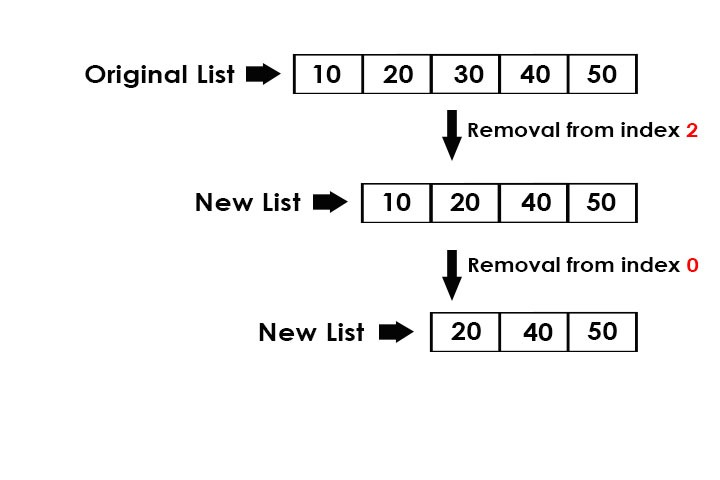# Python | Print list after removing element at given index

Given an index, remove the element at that index from the list and print the new list.Examples:

```Input : list = [10, 20, 30, 40, 50]
index = 2
Output : [10, 20, 40, 50]

Input : list = [10, 20, 40, 50]
index = 0
Output : [20, 40, 50]
```

## Recommended: Please try your approach on {IDE} first, before moving on to the solution.

Method 1: Traversal of list

Using traversal in the list, append all the index values except the given index to a new list and then print the new list. For this we will require a new list where we can append all the values except the given index value.

Below is the Python3 implementation of the above approach

 `# Python3 program to remove the index  ` `# element from the list  ` `# using traversal  ` ` `  `def` `remove(list1, pos): ` `    ``newlist ``=` `[] ` ` `  `    ``# traverse in the list ` `    ``for` `x ``in` `range``(``len``(list1)): ` `         `  `        ``# if index not equal to pos ` `        ``if` `x !``=` `pos: ` `            ``newlist.append(list1[x])  ` `    ``print``(``*``newlist)   ` ` `  `# driver code ` `list1 ``=` `[``10``, ``20``, ``30``, ``40``, ``50``] ` `pos ``=` `2` `remove(list1, pos) `

[/sourcecode]
Output:

```10 20 40 50
```

Method 2: pop()

pop() function helps us to pop the value at any desired position that is passed in the parameter, if nothing is passed in the parameter, then it removes the last index value.

Below is the Python3 implementation of the above approach:

 `# Python3 program to remove the index  ` `# element from the list  ` `# using pop() ` ` `  `def` `remove(list1, pos): ` ` `  `    ``# pop the element at index = pos ` `    ``list1.pop(pos)  ` `    ``print``(``*``list1) ` `     `  `     `  `# driver code ` `list1 ``=` `[``10``, ``20``, ``30``, ``40``, ``50``] ` `pos ``=` `2` `remove(list1, pos) `

Output:

```10 20 40 50
```

Method 3: del function

del function can be used to remove any element at any given position. If -1 or -2 is given in the [] brackets, then it deletes the last and second last element respectively.

Below is the Python3 implementation of the above approach:

 `# Python3 program to remove the index element ` `# from the list using del ` ` `  `def` `remove(list1, pos): ` ` `  `    ``# delete the element at index = pos ` `    ``del` `list1[pos]  ` `    ``print``(``*``list1) ` `     `  `     `  `# driver code ` `list1 ``=` `[``10``, ``20``, ``30``, ``40``, ``50``] ` `pos ``=` `2` `remove(list1, pos) `

Output:

```10 20 40 50
```

My Personal Notes arrow_drop_upCheck out this Author's contributed articles.

If you like GeeksforGeeks and would like to contribute, you can also write an article using contribute.geeksforgeeks.org or mail your article to contribute@geeksforgeeks.org. See your article appearing on the GeeksforGeeks main page and help other Geeks.

Please Improve this article if you find anything incorrect by clicking on the "Improve Article" button below.

Article Tags :
Practice Tags :

2

Please write to us at contribute@geeksforgeeks.org to report any issue with the above content.